Courses

# Subjective Type Questions: Aldehydes, Ketones & Carboxylic Acids- 2 | JEE Advanced Notes | EduRev

## JEE : Subjective Type Questions: Aldehydes, Ketones & Carboxylic Acids- 2 | JEE Advanced Notes | EduRev

The document Subjective Type Questions: Aldehydes, Ketones & Carboxylic Acids- 2 | JEE Advanced Notes | EduRev is a part of the JEE Course Class 12 Chemistry 35 Years JEE Mains &Advance Past yr Paper.
All you need of JEE at this link: JEE

Q.16. An organic compound (A) on treatment with acetic acid in the presence of sulphuric acid produces an ester (B), (A) on mild oxidation gives (C), (C) with 50% potassium hydroxide followed by acidification with dilute hydrochloric acid generates (A) and (D), (D) with phosphorus pentachloride followed by reaction with ammonia gives (E), (E) on dehydration produces hydrocyanic acid. Identify the compounds A, B, C, D and E.              (1987 - 5 Marks)

Ans.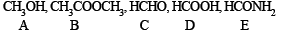Solution. TIPS/Formulae :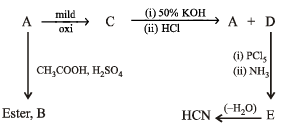The above reactions lead to following conclusions.

(i) Reaction of A with CH3COOH in presence of H2SO4 to form ester B indicates that A is an alcohol.
(ii) Reaction of C with 50% KOH followed by acidification to give alcohol A and the compound D seems to be the Cannizzaro reaction. Hence C must be an aldehyde and D must be an acid. The nature of C as aldehyde is again in consistent with the fact that it is obtained by the mild oxidation of A which has been established as an alcohol.
(iii) Structure of acid D is established by its given facts.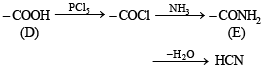Formation of HCN by the dehydration of E establishes that E is HCONH2 and hence D is HCOOH.

(iv) Thus the alcohol A produced along with HCOOH during Cannizzaro reaciton of C must be CH3OH and hence C must be HCHO.

Thus the various compounds are as below :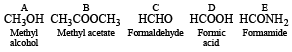Q.17. Complete the following reactions :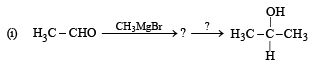(1988 - 1 Marks)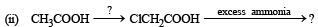(1988 - 1 Marks)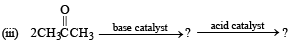(1988 - 1 Marks)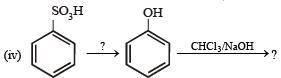(1988 - 1 Marks)

Solution.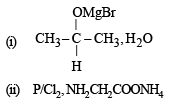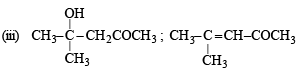(iv)  (i) fuse with NaOH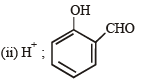Q.18. A hydrocarbon A (molecular formula C5H10) yields 2-methylbutane on catalytic hydrogenation. A adds HBr (in accordance with Markownikoff’s rule) to form a compound B which on reaction with silver hydroxide forms an alcohol C, C5H12O. Alcohol C on oxidation gives a ketone D. Deduce the structures of A, B, C and D and show the reactions involved.             (1988 - 5 Marks)

Ans.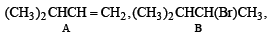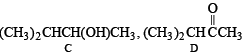Solution. TIPS/Formulae :

For this type of problem, students are advised to summarise the whole problem in the form of reactions.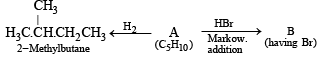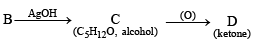Let us draw some conclusions from the above set of reactions.

(i) The molecular formula C5H10 (CnH2n) for A indicates that it is an alkene having one double bond.
(ii) Since the alcohol C on oxidation gives a ketone D, C must be a secondary alcohol and hence B must be a secondary bromide.
(iii) The structure of 2-methylbutane, the hydrogenated product of A, indicates that the secondary bromide must have following structure.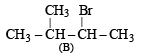(iv) Thus the corresponding olefin A must have structure A which on Markownikoff addition of HBr gives the bromide B, the other possible alkene A' will not give B when HBr is addd on it according to Markownikoff rule.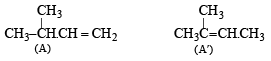Thus the reaction involved can be represented as below: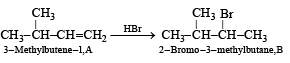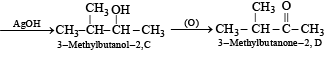Q.19. A ketone ‘A’ wh ich undergoes haloform reaction gives compound B on reduction. B on heating with sulphuric acid gives compound C, which forms monoozonide D, D on hydrolysis in presence of zinc dust gives only acetaldehyde. Identify A, B and C. Write down the reactions involved.  (1989 - 4 Marks)

Ans.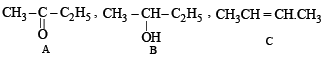Solution. The compound A, a ketone, undergoes haloform reaction.

Thus, it must contain CH3CO group.

The compound C gives mono-ozonide D, which shows that the compound C contains a double bond. Since the hydrolysis of D gives only acetaldehyde, the compound C would be an alkene having four carbon atoms,

i.e. CH3 – CH = CH – CH3 (butene-2).

The compound B is obtained by the reduction of compound A (which contains CH3CO group). Hence, the compound B would be an alcohol, which on heating with H2SO4 gives (C). Hence B and A would be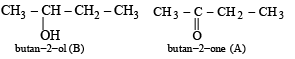The reactions involved :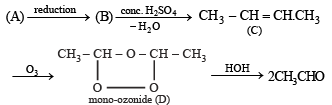Q.20. The sodium salt of a carboxylic acid, A, was produced by passing a gas, B, into an aqueous solution of caustic alkali at an elevated temperature and pressure. A, on heating in presence of sodium hydroxide followed by treatment with sulphuric acid gave a dibasic acid, C. A sample of 0.4 g of acid C, on combustion gave 0.08 g of water and 0.39 g of carbon dioxide. The silver salt of the acid C weighing 1.0 g on ignition yielded 0.71 g of silver as residue. Identify A, B and C.  (1990 - 5 Marks)

Ans. TIPS/Formulae :

The given set of reactions can be represented as below :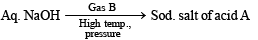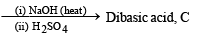Calculation of molecular formula of C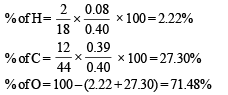By usual method, empirical formula of acid C = CHO2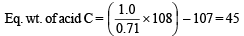Mol. wt. of acid C = 45 × 2 = 90
∴ Mol. formula of C = C2H2O4
Since it is dicarboxylic acid, it must have two –COOH groups.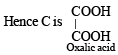Going back, compound C must be produced from sodium oxalate which in turn is produced from sodium formate.
Hence A is formic acid and B is CO2. Thus the complete series of reactions can be written as below.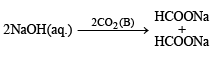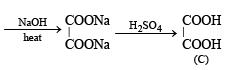Q.21. Compound A (C6H12O2) on reduction with LiAlH4 yielded two compounds B and C. The compound B on oxidation gave D, which on treatment with aqueous alkali and subsequent heating furnished E. The latter on catalytic hydrogenation gave C. The compound D was oxidized further to give F which was found to be a monobasic acid (molecular weight = 60.0). Deduce the structures of A, B, C, D and E.              (1990 - 4 Marks)

Ans.  C3H7COOC2H5, C2H5OH, C4H9OH, CH3CHO

Solution. TIPS/FORMULAE :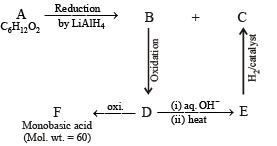Molecular weight of the monobasic acid (RCOOH) indicates that the R– should be CH3– i.e., acid F should be acetic acid (CH3COOH, mol. wt. 15+45). Thus compound D must be acetaldehyde CH3CHO, and compound B which on oxidation gives CH3CHO must be ethanol, CH3CH2OH.
Acetaldehyde (D) on treating with aqueous alkali will undergo aldol condensation.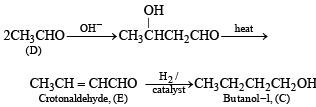Nature of A. Thus it is evident that reduction of A with LiAlH4 gives two alcohols; B (ethanol) and C (butanol). Hence A must be an ester i.e., ethyl butanoate (CH3CH2CH2COOC2H5).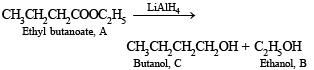Q.22. An organic compound containing C, H and O exists in two isomeric forms A and B. An amount of 0.108 g of one of the isomers gives on combustion 0.308 g of CO2 and 0.072 g of H2O. A is insoluble in NaOH and NaHCO3 while B is soluble in NaOH. A reacts with conc. HI to give compounds C and D. C can be separated from D by ethanolic AgNO3 solution and D is soluble in NaOH. B reacts readily with bromine water to give compound E of molecular formula, C7H5OBr3.
Identify, A, B, C, D and E with justification and give their structures.             (1991 - 6 Marks)

Ans.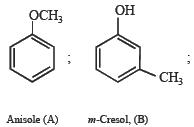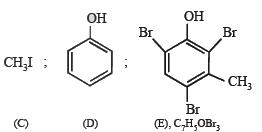Solution. Empirical formula of A and B.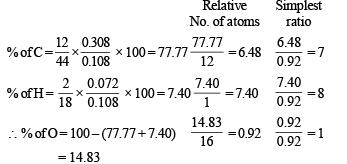∴ Empirical formula of A and B = C7H8O
Nature of (A) : Since A is insoluble in NaOH and NaHCO3, it can’t have –OH and –COOH groups. Further the reaction of A with conc. HI to give compounds C and D separable by means of ammonical AgNO3 and solubility of D in NaOH indicates that C and D are alkyl halide and phenol respectively. Thus A is an ether i.e. it is C6H5.O.CH3 which explains all the given reactions.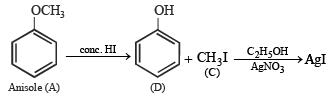Nature of (B) : Solubility of B (C7H8O) in NaOH indicates that it is a phenol which is further confirmed by its reaction with bromine water to give compound E of molecular formula, C7H5OBr3. Further bromination of B to give tribromo product indicates that it is m-cresol.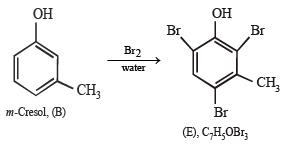Q.23. (i)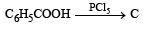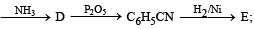Identify C, D and E.                                                                       (1991 - 2 Marks)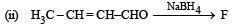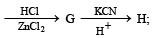Identify F, G and H.                                         (1991 - 2 Marks)

Solution.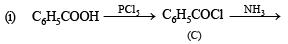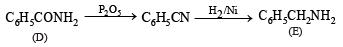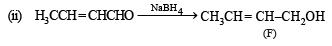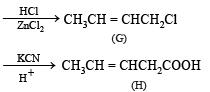Q.24. Compound ‘X’, containing chlorine on treatment with strong ammonia gives a solid ‘Y’ which is free from chlorine. ‘Y’ analysed as C = 49.31%, H = 9.59% and N = 19.18% and reacts with Br2 and caustic soda to give a basic compound ‘Z’. ‘Z’ reacts with HNO2 to give ethanol. Suggest structures for ‘X’, ‘Y’ and ‘Z’.      (1992 - 1 Mark)

Ans.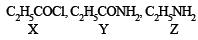Solution. For empirical formula of (Y)

 Element % Relative no. of atoms Simplestratio C 49.31 4.10 3 H 9.59 9.59 7 N 19.18 1.37 1 O 21.92 1.37 1

∴ Empirical formula of (Y) is C3H7NO.

(Y) reacts with Br2 and NaOH to give (Z) and (Z) reacts with HNO2 to give ethanol and thus (Y) seems to have —CONH2 group.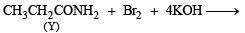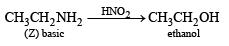Y is formed from (X) having Cl on treatment with NH3 and so (X) is CH3CH2COCl i.e. propanoyl chloride.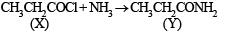Q.25. An organic compound ‘A’ on treatment with ethyl alcohol gives a carboxylic acid ‘B’ and compound ‘C’. Hydrolysis of ‘C’ under acidic conditions gives ‘B’ and ‘D’. Oxidation of ‘D’ with KMnO4 also gives ‘B’. ‘B’ on heating with Ca(OH)2 gives ‘E’ (molecular formula, C3H6O). ‘E’ does not give Tollent’s test and does not reduce Fehling’s solution but forms a 2,4-dinitrophenylhydrazone. Identify ‘A’, ‘B’, ‘C’, ‘D’ and ‘E’.An organic compound ‘A’ on treatment with ethyl alcohol gives a carboxylic acid ‘B’ and compound ‘C’. Hydrolysis of ‘C’ under acidic conditions gives ‘B’ and ‘D’. Oxidation of ‘D’ with KMnO4 also gives ‘B’. ‘B’ on heating with Ca(OH)2 gives ‘E’ (molecular formula, C3H6O). ‘E’ does not give Tollent’s test and does not reduce Fehling’s solution but forms a 2,4-dinitrophenylhydrazone. Identify ‘A’, ‘B’, ‘C’, ‘D’ and ‘E’.                            (1992 - 3 Marks)

Ans.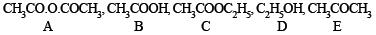Solution. TIPS/Formulae :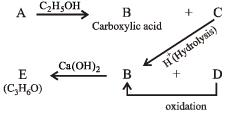(i) Since E (C3H6O) forms a 2, 4-dinitrophenylhydrazone but does not reduce Tollen’s reagent and Fehling solution, it must be a ketone, CH3.CO.CH3.
(ii) The compound E (established as ketone) is obtained by heating compound B with Ca(OH)2, B must be CH3COOH.
(iii) Compound B is obtained by the oxidation of D, the latter must be ethyl alcohol, C2H5OH and hence C must be ethyl acetate, CH3COOC2H5.
(iv) Since compound A when treated with ethyl alcohol gives acetic acid (B) and ethyl acetate (C), it must be acetic anhydride.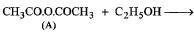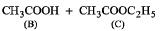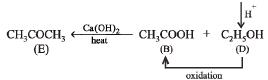Q.26. Arrange the following in increasing order of expected enol content      (1992 - 1 Mark)

CH3COCH2CHO3 CH3COCH3, CH3CHO, CH3COCH2COCH3

Ans.  CH3CHO < CH3COCH3 < CH3COCH2CHO < CH3COCH2COCH3

Solution. CH3CHO < CH3COCH3 < CH3COCH2COCH3 < CH3COCH2CHO

Q.27. In the following reactions identify the compounds A, B, C and D.                (1994 - 1 × 4 = 4 Marks)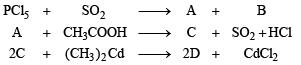Ans.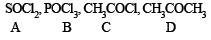Solution.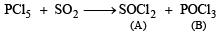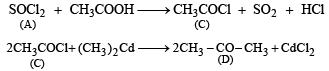Q.28. When gas A is passed through dry KOH at low temperature, a deep red coloured compound B and a gas C are obtained. The gas A, on reaction with but-2-ene, followed by treatment with Zn/H2O yields acetaldehyde. Identify A, B and C.              (1994 - 3 Marks)

Ans.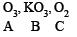Solution. TIPS/Formulae :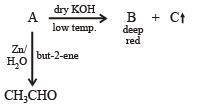The reaction of gas (A) with but-2-ene followed by treatment with Zn/H2O gives CH3CHO. This shows that the gas (A) is ozone (O3).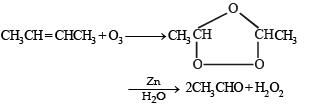Reaction of ozone with KOH.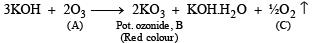Q.29. An organic compound A, C8H6, on treatment with dilute sulphuric acid containing mercuric sulphate gives a compound B, which can also be obtained from a reaction of benzene with an acid chloride in the presence of anhydrous aluminium chloride. The compound B, when treated with iodine in aqueous KOH, yields C and a yellow compound D. Identify A, B, C and D with justification. Show how B is formed from A.                       (1994 - 3 Marks)

Ans.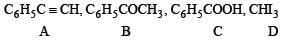Solution. TIPS/Formulae :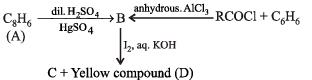(i) Formation of (B) from benzene and acid chloride in presence of anhydrous AlCl3 (Friedel-Craft reaction) indicates that it is a ketone, C6H5COR.

(ii) Further th e keton e (B) reacts with alkaline iodine forming yellow compound (D) (haloform reaction). This indicates that one of the alkyl groups in ketone B is – CH3. Hence it should be C6H5.CO.CH,.

(iii) Since ketone (B) is also formed from the hydrocarbon C8H6 (A) by reaction with dil. H2SO4 and HgSO,, the hydrocabon (A) must have an acetylenic hydrogen atom, i.e. ≡ C – H grouping. Hence (A) must be C6H5C ≡ CH.

Thus compounds (A) to (D) are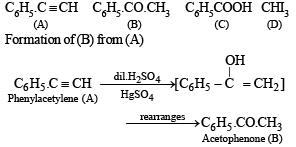Q.30. Which of the following carboxylic acids under goes decarboxylation easily? Explain briefly.               (1995 - 2 Marks)

(i) C6H5–CO–CH2–COOH
(ii) C6H5–CO–COOH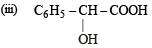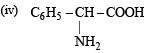Solution. (i) β-Keto acids are unstable and undergo decarboxylation most readily.

Offer running on EduRev: Apply code STAYHOME200 to get INR 200 off on our premium plan EduRev Infinity!

68 docs|36 tests

,

,

,

,

,

,

,

,

,

,

,

,

,

,

,

,

,

,

,

,

,

,

,

,

;